# Finding the path from one vertex to rest using BFS

Given an adjacency list representation of a directed graph, the task is to find the path from source to every other node in the graph using BFS.

Examples:

```Input: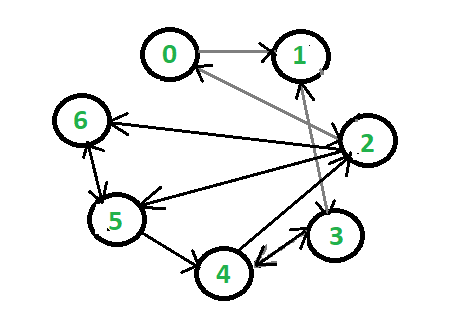Output:
0 <- 2
1 <- 0 <- 2
2
3 <- 1 <- 0 <- 2
4 <- 5 <- 2
5 <- 2
6 <- 2
```

## Recommended: Please try your approach on {IDE} first, before moving on to the solution.

Approach: In the images shown below:

• que[] array stores the vertices reached and we will enqueue a vertex only if it has not been visited and dequeue it once all its child node have been considered.
• In order to distinguish whether the node has been visited or not we will put 1 in visited[] array at the respective index to signify it has been visited and if at given index 0 is present it will signify that it has not been visited.
• Parent array is to store the parent node of each vertex. For ex. In case of 0 connected to 2, 2 will be the parent node of 0 and we will put 2 at the index 0 in the parent array.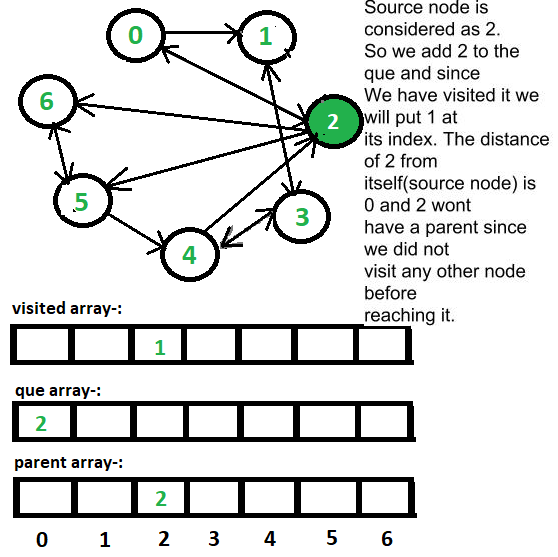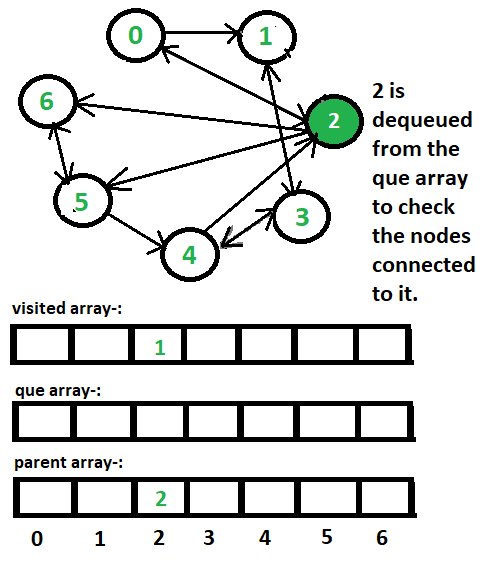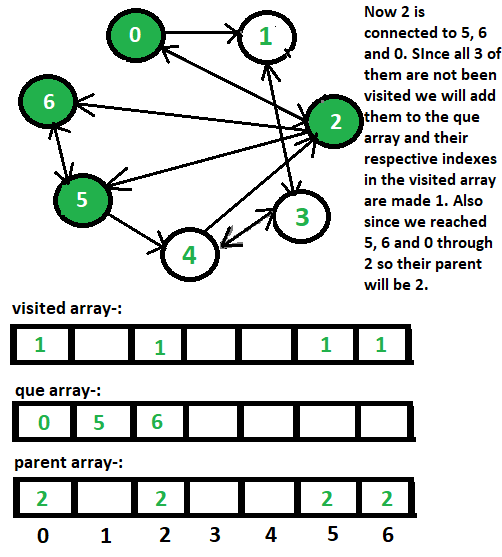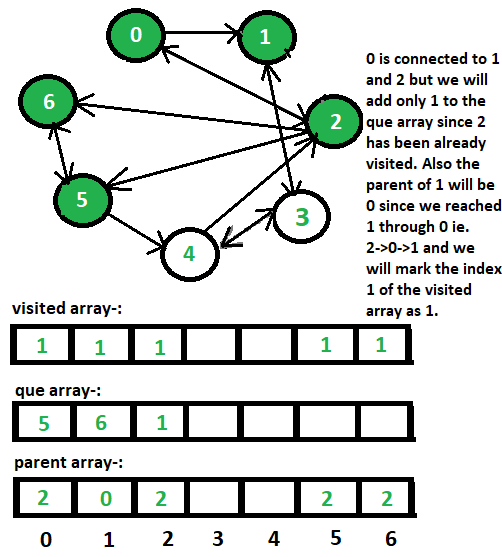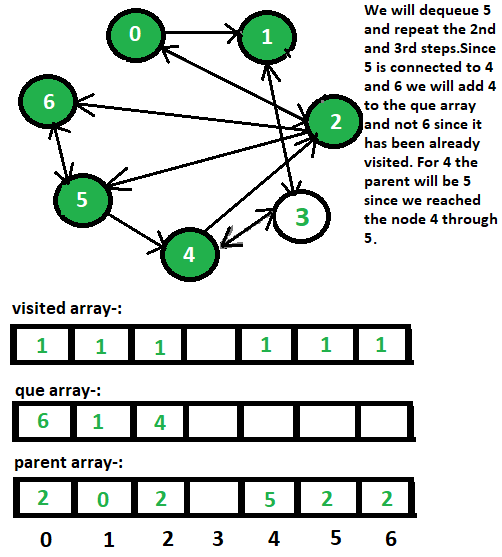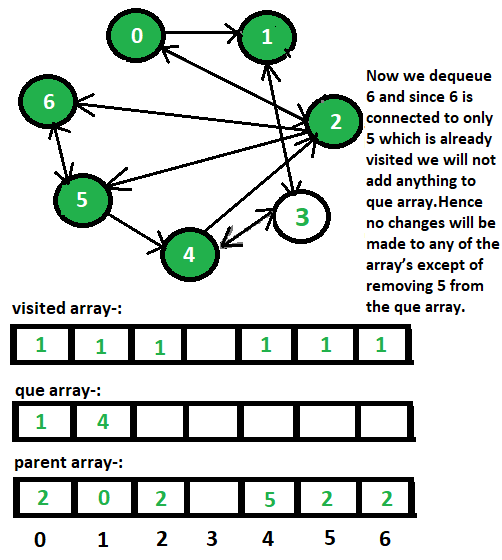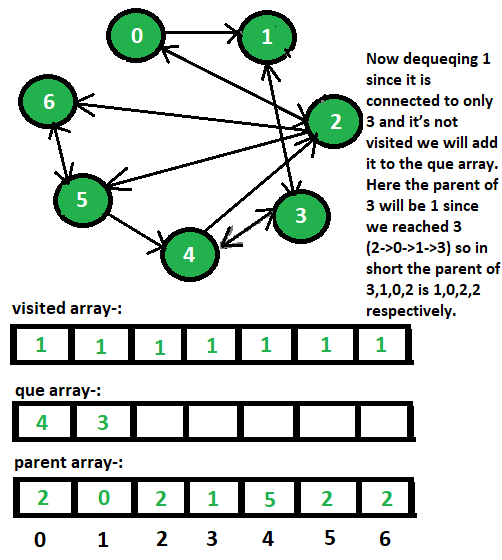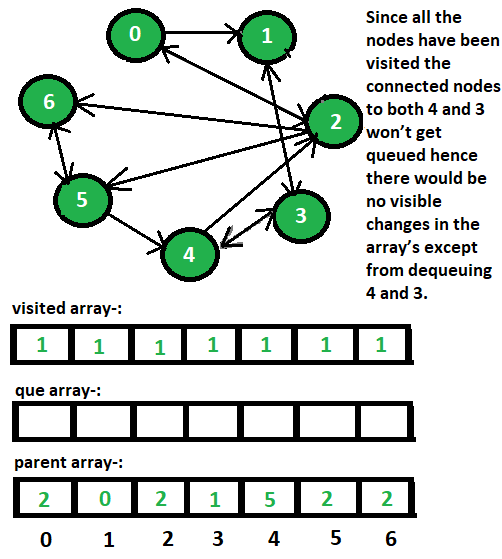Below is the implementation of the above approach:

## Java

 `// Java implementation of the approach ` `import` `java.util.ArrayList; ` `import` `java.util.Arrays; ` `import` `java.util.List; ` ` `  `class` `GFG { ` ` `  `    ``// Function to print the path from ` `    ``// source (s) to destination (d) ` `    ``static` `void` `print(``int` `parent[], ``int` `s, ``int` `d) ` `    ``{ ` `        ``// The while loop will stop only when the ` `        ``// destination and the source node become equal ` `        ``while` `(s != d) { ` ` `  `            ``// Print the destination and store the parent ` `            ``// of the node in the destination since parent ` `            ``// stores the node through which ` `            ``// the current node has been reached ` `            ``System.out.print(d + ``" <- "``); ` `            ``d = parent[d]; ` `        ``} ` ` `  `        ``System.out.println(d); ` `    ``} ` ` `  `    ``// Finding Path using BFS ALgorithm ` `    ``static` `void` `bfs(List > adjList, ``int` `source, ``int` `n) ` `    ``{ ` `        ``int` `parent[] = ``new` `int``[n]; ` `        ``int` `que[] = ``new` `int``[n]; ` `        ``Arrays.fill(parent, ``0``); ` `        ``Arrays.fill(que, ``0``); ` ` `  `        ``int` `front = -``1``, rear = -``1``; ` `        ``int` `visited[] = ``new` `int``[n]; ` `        ``Arrays.fill(visited, ``0``); ` `        ``visited = ``1``; ` `        ``parent = source; ` ` `  `        ``// To add any non visited node we will increment the rear ` `        ``// and add that vertex to the end of the array (enqueuing) ` `        ``que[++rear] = source; ` ` `  `        ``int` `k; ` ` `  `        ``// The loop will continue till the rear and front are equal ` `        ``while` `(front != rear) { ` ` `  `            ``// Here Dequeuing is nothing but to increment the front int ` `            ``k = que[++front]; ` `            ``List list = adjList.get(k); ` `            ``for` `(``int` `i = ``0``; i < list.size(); i++) { ` `                ``int` `j = list.get(i); ` `                ``if` `(visited[j] == ``0``) { ` `                    ``que[++rear] = j; ` `                    ``visited[j] = ``1``; ` `                    ``parent[j] = k; ` `                ``} ` `            ``} ` `        ``} ` ` `  `        ``// Print the path from source to every other node ` `        ``for` `(k = ``0``; k < n; k++) ` `            ``print(parent, source, k); ` `    ``} ` ` `  `    ``// Driver code ` `    ``public` `static` `void` `main(String args[]) ` `    ``{ ` ` `  `        ``// Adjacency list representation of the graph ` `        ``List > adjList = ``new` `ArrayList<>(); ` ` `  `        ``// Vertices 1 and 2 have an incoming edge ` `        ``// from vertex 0 ` `        ``List tmp = ``new` `ArrayList(Arrays.asList(``1``, ``2``)); ` `        ``adjList.add(tmp); ` ` `  `        ``// Vertex 3 has an incoming edge from vertex 1 ` `        ``tmp = ``new` `ArrayList(Arrays.asList(``3``)); ` `        ``adjList.add(tmp); ` ` `  `        ``// Vertices 0, 5 and 6 have an incoming ` `        ``// edge from vertex 2 ` `        ``tmp = ``new` `ArrayList(Arrays.asList(``0``, ``5``, ``6``)); ` `        ``adjList.add(tmp); ` ` `  `        ``// Vertices 1 and 4 have an incoming edge ` `        ``// from vertex 3 ` `        ``tmp = ``new` `ArrayList(Arrays.asList(``1``, ``4``)); ` `        ``adjList.add(tmp); ` ` `  `        ``// Vertices 2 and 3 have an incoming edge ` `        ``// from vertex 4 ` `        ``tmp = ``new` `ArrayList(Arrays.asList(``2``, ``3``)); ` `        ``adjList.add(tmp); ` ` `  `        ``// Vertices 4 and 6 have an incoming edge ` `        ``// from vertex 5 ` `        ``tmp = ``new` `ArrayList(Arrays.asList(``4``, ``6``)); ` `        ``adjList.add(tmp); ` ` `  `        ``// Vertex 5 has an incoming edge from vertex 6 ` `        ``tmp = ``new` `ArrayList(Arrays.asList(``5``)); ` `        ``adjList.add(tmp); ` ` `  `        ``int` `n = adjList.size(); ` ` `  `        ``int` `source = ``2``; ` `        ``bfs(adjList, source, n); ` `    ``} ` `} `

## Python3

 `# Python3 implementation of the approach  ` ` `  `# Function to print the path from  ` `# src (s) to destination (d)  ` `def` `printfunc(parent, s, d):  ` `     `  `    ``# The while loop will stop only when  ` `    ``# the destination and the src node  ` `    ``# become equal  ` `    ``while` `s !``=` `d:  ` ` `  `        ``# Print the destination and store  ` `        ``# the parent of the node in the  ` `        ``# destination since parent stores ` `        ``# the node through which the current ` `        ``# node has been reached  ` `        ``print``(``str``(d) ``+` `" <-"``, end ``=` `" "``)  ` `        ``d ``=` `parent[d]  ` `         `  `    ``print``(d)  ` ` `  `# Finding Path using BFS ALgorithm  ` `def` `bfs(adjList, src, n):  ` `     `  `    ``parent ``=` `[``0``] ``*` `(n)  ` `    ``que ``=` `[``0``] ``*` `(n)  ` `     `  `    ``front, rear ``=` `-``1``, ``-``1` `    ``visited ``=` `[``0``] ``*` `(n)  ` `    ``visited[src] ``=` `1` `    ``parent[src] ``=` `src ` ` `  `    ``# To add any non visited node we will  ` `    ``# increment the rear and add that vertex  ` `    ``# to the end of the array (enqueuing)  ` `    ``rear ``+``=` `1` `    ``que[rear] ``=` `src  ` ` `  `    ``# The loop will continue till the rear  ` `    ``# and front are equal  ` `    ``while` `front !``=` `rear:  ` ` `  `        ``# Here Dequeuing is nothing but to ` `        ``# increment the front int  ` `        ``front ``+``=` `1` `        ``k ``=` `que[front]  ` `        ``List` `=` `adjList[k]  ` `        ``for` `i ``in` `range``(``0``, ``len``(``List``)):  ` `            ``j ``=` `List``[i] ` `             `  `            ``if` `visited[j] ``=``=` `0``: ` `                ``rear ``+``=` `1` `                ``que[rear] ``=` `j  ` `                ``visited[j] ``=` `1` `                ``parent[j] ``=` `k  ` `                 `  `    ``# Print the path from src to every  ` `    ``# other node  ` `    ``for` `k ``in` `range``(``0``, n):  ` `        ``printfunc(parent, src, k)  ` `     `  `# Driver code  ` `if` `__name__ ``=``=` `"__main__"``:  ` ` `  `    ``# Adjacency list representation ` `    ``# of the graph  ` `    ``adjList ``=` `[]  ` ` `  `    ``# Vertices 1 and 2 have an incoming edge  ` `    ``# from vertex 0  ` `    ``adjList.append([``1``, ``2``])  ` ` `  `    ``# Vertex 3 has an incoming edge  ` `    ``# from vertex 1  ` `    ``adjList.append([``3``])  ` ` `  `    ``# Vertices 0, 5 and 6 have an incoming  ` `    ``# edge from vertex 2  ` `    ``adjList.append([``0``, ``5``, ``6``])  ` ` `  `    ``# Vertices 1 and 4 have an incoming edge  ` `    ``# from vertex 3  ` `    ``adjList.append([``1``, ``4``])  ` ` `  `    ``# Vertices 2 and 3 have an incoming edge  ` `    ``# from vertex 4  ` `    ``adjList.append([``2``, ``3``])  ` ` `  `    ``# Vertices 4 and 6 have an incoming edge  ` `    ``# from vertex 5  ` `    ``adjList.append([``4``, ``6``])  ` ` `  `    ``# Vertex 5 has an incoming edge  ` `    ``# from vertex 6  ` `    ``adjList.append([``5``])  ` ` `  `    ``n ``=` `len``(adjList)  ` ` `  `    ``src ``=` `2` `    ``bfs(adjList, src, n)  ` `     `  `# This code is contributed by Rituraj Jain `

Output:

```0 <- 2
1 <- 0 <- 2
2
3 <- 1 <- 0 <- 2
4 <- 5 <- 2
5 <- 2
6 <- 2
```

Attention reader! Don’t stop learning now. Get hold of all the important DSA concepts with the DSA Self Paced Course at a student-friendly price and become industry ready.

My Personal Notes arrow_drop_upCheck out this Author's contributed articles.

If you like GeeksforGeeks and would like to contribute, you can also write an article using contribute.geeksforgeeks.org or mail your article to contribute@geeksforgeeks.org. See your article appearing on the GeeksforGeeks main page and help other Geeks.

Please Improve this article if you find anything incorrect by clicking on the "Improve Article" button below.

Improved By : rituraj_jain